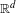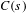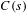Hostname: page-component-5db6c4db9b-fdz9p Total loading time: 0 Render date: 2023-03-25T05:28:02.040Z Has data issue: true Feature Flags: { "useRatesEcommerce": false } hasContentIssue true

# Random affine code tree fractals and Falconer–Sloan condition

Published online by Cambridge University Press:  15 December 2014

## Abstract

We calculate the almost sure dimension for a general class of random affine code tree fractals in$\mathbb{R}^{d}$. The result is based on a probabilistic version of the Falconer–Sloan condition$C(s)$ introduced in Falconer and Sloan [Continuity of subadditive pressure for self-affine sets. Real Anal. Exchange 34 (2009), 413–427]. We verify that, in general, systems having a small number of maps do not satisfy condition$C(s)$. However, there exists a natural number$n$ such that for typical systems the family of all iterates up to level$n$ satisfies condition$C(s)$.

Type
Research Article
Information
Ergodic Theory and Dynamical Systems , August 2016 , pp. 1516 - 1533

## Access options

Get access to the full version of this content by using one of the access options below. (Log in options will check for institutional or personal access. Content may require purchase if you do not have access.)

## References

Barnsley, M., Hutchinson, J. E. and Stenflo, Ö.. A fractal valued random iteration algorithm and fractal hierarchy. Fractals 13 (2005), 111146.CrossRefGoogle Scholar
Barnsley, M., Hutchinson, J. E. and Stenflo, Ö.. V-variable fractals: fractals with partial self similarity. Adv. Math. 218 (2008), 20512088.CrossRefGoogle Scholar
Barnsley, M., Hutchinson, J. E. and Stenflo, Ö.. V-variable fractals: dimension results. Forum Math. 24 (2012), 445470.CrossRefGoogle Scholar
Barral, J. and Feng, D.-J.. Multifractal formalism for almost all self-affine measures. Comm. Math. Phys. 318 (2013), 473504.CrossRefGoogle Scholar
Falconer, K. J.. The Hausdorff dimension of self-affine fractals. Math. Proc. Cambridge Philos. Soc. 103 (1988), 339350.CrossRefGoogle Scholar
Falconer, K. J.. Sub-self-similar sets. Trans. Amer. Math. Soc. 347 (1995), 31213129.CrossRefGoogle Scholar
Falconer, K. J.. Fractal Geometry, 2nd edn. John Wiley, Chichester, 2003.CrossRefGoogle Scholar
Falconer, K. J. and Miao, J.. Dimensions of self-affine fractals and multifractals generated by upper triangular matrices. Fractals 15 (2007), 289299.CrossRefGoogle Scholar
Falconer, K. J. and Miao, J.. Random subsets of self-affine fractals. Mathematika 56 (2010), 6176.CrossRefGoogle Scholar
Falconer, K. J. and Sloan, A.. Continuity of subadditive pressure for self-affine sets. Real Anal. Exchange 34 (2009), 413427.CrossRefGoogle Scholar
Feng, D.-J.. Lyapunov exponents for products of matrices and multifractal analysis. Part II: General matrices. Israel J. Math. 170 (2009), 355394.CrossRefGoogle Scholar
Feng, D.-J. and Shmerkin, P.. Non-conformal repellers and the continuity of pressure for matrix cocycles. Geom. Funct. Anal. 24 (2014), 11011128.CrossRefGoogle Scholar
Järvenpää, E., Järvenpää, M., Käenmäki, A., Koivusalo, H., Stenflo, Ö. and Suomala, V.. Dimensions of random affine code tree fractals. Ergod. Th. & Dynam. Sys. 34 (2014), 854875.CrossRefGoogle Scholar
Jordan, T., Pollicott, M. and Simon, K.. Hausdorff dimension for randomly perturbed self affine attractors. Comm. Math. Phys. 270 (2007), 519544.CrossRefGoogle Scholar
Käenmäki, A. and Shmerkin, P.. Overlapping self-affine sets of Kakeya type. Ergod. Th. & Dynam. Sys. 29 (2009), 941965.CrossRefGoogle Scholar
Kechris, A.. Classical Descriptive Set Theory. Springer, New York, 1995.CrossRefGoogle Scholar
Mauldin, R. D. and Urbański, M.. Graph Directed Markov Systems: Geometry and Dynamics of Limit Sets (Cambridge Tracts in Mathematics, 148) . Cambridge University Press, Cambridge, 2003.CrossRefGoogle Scholar
Przytycki, F. and Urbański, M.. On the Hausdorff dimension of some fractal sets. Studia Math. 93 (1989), 155186.CrossRefGoogle Scholar
Roy, M. and Urbański, M.. Random graph directed Markov systems. Discrete Contin. Dyn. Syst. 30 (2011), 261298.Google Scholar
Solomyak, B.. Measure and dimensions for some fractal families. Math. Proc. Cambridge Philos. Soc. 124 (1998), 531546.CrossRefGoogle Scholar